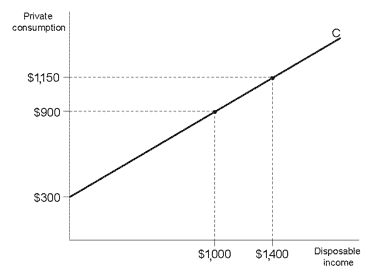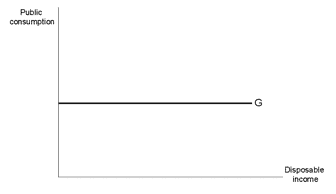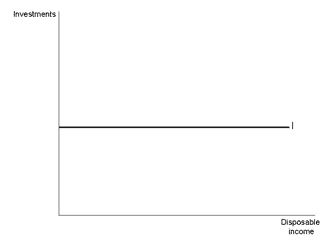# Keynes’ Explanation Concerning Curves

The explanation applies to a closed economy aggregate demand (denoted by E) and is composed of three elements:

1. Private consumption (buying goods from the private sector) is denoted by C = consumption.

2. Public consumption (receiving services from the state) is denoted by G = government.

3. Investments (buying investment goods) is denoted by I = investment.

The following mathematical relationship exists: E=C+G+I

## Presenting Demand Factors at the Individual Level

### Private consumption – C

As a person’s disposable income increases, he consumes more. The C curve in Diagram 11.6 reflects this relationship.

Diagram 11.6

The relationship between disposable income and consumptionFor the sake of simplicity, we will make two assumptions:

1. The C curve is a straight line that rises whenever disposable income rises. The significance of the straight line is that with each additional USD of disposable income, consumption rises by an equivalent amount. Example: \$0.60 is consumed for each additional USD.
2. Even when a person has no disposable income, he still consumes to a lesser degree.### Public consumption – G

For the sake of simplicity, Keynes assumes that the amount of public consumption does not vary with a rise in disposable income.

In other words, the level of services that a person receives from the state does not depend on his disposable income (as illustrated in Diagram 11.7).

Diagram 11.7

Public Consumption### Investment – I

Keynes once again assumes that I is a straight line, so the amount of investment does not vary with the increase in disposable income, as illustrated in Diagram 11.8.

Diagram 11.8

Investment## Displaying aggregate demand Factors at the macroeconomic level

Demand in every factor includes the total demand of all residents, and the demand curve of every factor resembles the demand curve at the individual level.

Diagram 11.9 presents aggregate demand E and each of its components – CG, and I – in separate curves.

Diagram 11.9

Layers of aggregate demand at the macroeconomic levelThe X axis in Diagram 11.9 represents GDP (and not disposable income), which is described at the level of individual demand. The reason is that the total income of all residents = GDP